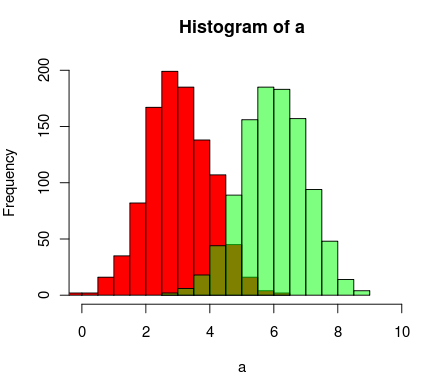## Transparent histograms redux

August 19th, 2010

The other day, I posted an R function that simulates transparency where histograms overlap. In the comments, Alice left this tip:

You could also use transparent colors, which could mean less programming effort:
e.g. col=rgb(0, 1, 0,0.5)) ..the 4th argument is the degree of transparency alpha

*smacks forehead* That’s a lot simpler than my solution. I didn’t know R supported transparency like that. So instead of using the function I wrote, I could have just done something like this:

```a=rnorm(1000, 3, 1) b=rnorm(1000, 6, 1) hist(a, xlim=c(0,10), col="red") hist(b, add=T, col=rgb(0, 1, 0, 0.5)```

And gotten the following output:Much simpler.